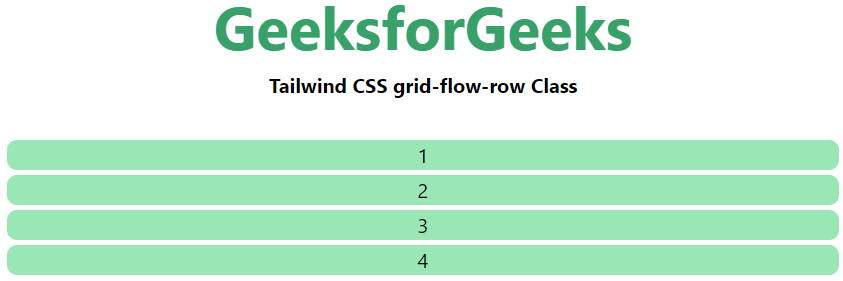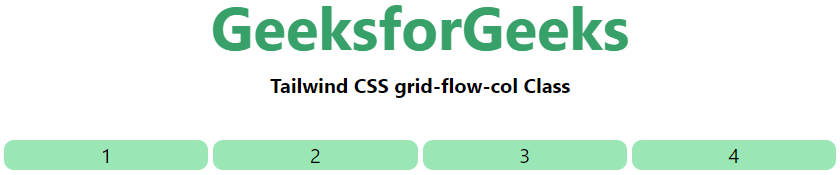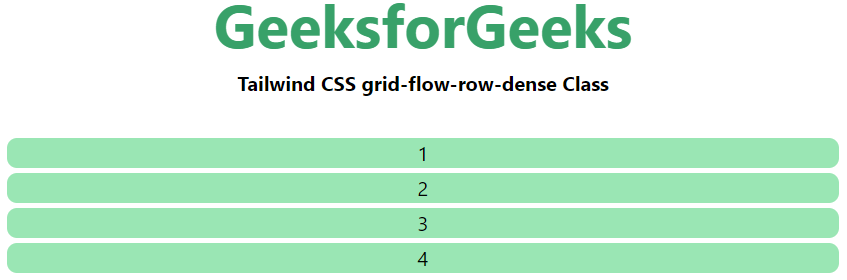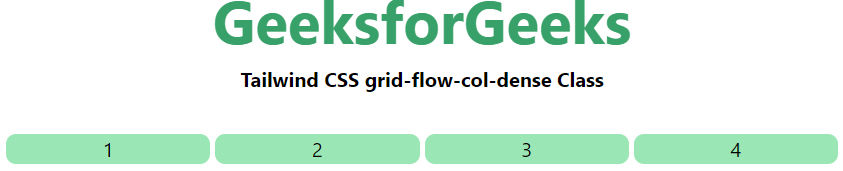# Tailwind CSS Grid Auto Flow

This class accepts more than one value in tailwind CSS all the properties are covered as in class form. It is the alternative of CSS grid-auto-flow property and it is used to specify how auto-placed items get flowed into the grid items using Tailwind CSS.

Grid Auto Flow:

• grid-flow-row
• grid-flow-col
• grid-flow-row-dense
• grid-flow-col-dense

grid-flow-row: Auto-placement algorithm place the items by filling each row in turn, adding new rows as necessary.

Syntax:

`<element class="grid-flow-row"> Contents... </element>`

Example:

## HTML

 `  ` `<``html``> ` ` `  `<``head``>  ` `    ``<``title``>Tailwind grid-flow-row Class  ` `    ``<``link` `href``= ` `"https://unpkg.com/tailwindcss@^1.0/dist/tailwind.min.css"`  `          ``rel``=``"stylesheet"``>  ` `  ` ` `  `<``body` `class``=``"text-center"``>  ` `    ``<``h1` `class``=``"text-green-600 text-5xl font-bold"``> ` `        ``GeeksforGeeks ` `    ``  ` ` `  `    ``<``b``>Tailwind CSS grid-flow-row Class  ` ` `  `    ``<``div` `class` `= ``"m-8 grid grid-flow-row gap-1"``>   ` `        ``<``div` `class` `= ``"bg-green-300 rounded-lg"``>1   ` `        ``<``div` `class` `= ``"bg-green-300 rounded-lg"``>2   ` `        ``<``div` `class` `= ``"bg-green-300 rounded-lg"``>3   ` `        ``<``div` `class` `= ``"bg-green-300 rounded-lg"``>4   ` `    ``   ` `  ` `   `  ``

Output:grid-flow-col: Auto-placement algorithm place the items by filling each column in turn, adding new columns as necessary.

Syntax:

`<element class="grid-flow-col"> Contents... </element>`

Example:

## HTML

 `  ` `<``html``> ` ` `  `<``head``>  ` `    ``<``title``>Tailwind grid-flow-col Class  ` `    ``<``link` `href``= ` `"https://unpkg.com/tailwindcss@^1.0/dist/tailwind.min.css"`  `          ``rel``=``"stylesheet"``>  ` `  ` ` `  `<``body` `class``=``"text-center"``>  ` `    ``<``h1` `class``=``"text-green-600 text-5xl font-bold"``> ` `        ``GeeksforGeeks ` `    ``  ` ` `  `    ``<``b``>Tailwind CSS grid-flow-col Class  ` ` `  `    ``<``div` `class` `= ``"m-8 grid grid-flow-col gap-1"``>   ` `        ``<``div` `class` `= ``"bg-green-300 rounded-lg"``>1   ` `        ``<``div` `class` `= ``"bg-green-300 rounded-lg"``>2   ` `        ``<``div` `class` `= ``"bg-green-300 rounded-lg"``>3   ` `        ``<``div` `class` `= ``"bg-green-300 rounded-lg"``>4   ` `    ``   ` `  ` `   `  ``

Output:grid-flow-row-dense: Specifying that the auto-placement algorithm uses a “dense” packing algorithm for the column.

Syntax:

`<element class="grid-flow-row-dense"> Contents... </element>`

Example:

## HTML

 ` ` `<``html``> ` `  `  `<``head``>  ` `    ``<``title``>Tailwind grid-flow-row-dense Class  ` `    ``<``link` `href``= ` `"https://unpkg.com/tailwindcss@^1.0/dist/tailwind.min.css"`  `          ``rel``=``"stylesheet"``>  ` `  ` ` `  `<``body` `class``=``"text-center"``>  ` `    ``<``h1` `class``=``"text-green-600 text-5xl font-bold"``> ` `        ``GeeksforGeeks ` `    ``  ` ` `  `    ``<``b``>Tailwind CSS grid-flow-row-dense Class  ` ` `  `    ``<``div` `class` `= ``"m-8 grid grid-flow-row-dense gap-1"``>   ` `        ``<``div` `class` `= ``"bg-green-300 rounded-lg"``>1   ` `        ``<``div` `class` `= ``"bg-green-300 rounded-lg"``>2   ` `        ``<``div` `class` `= ``"bg-green-300 rounded-lg"``>3   ` `        ``<``div` `class` `= ``"bg-green-300 rounded-lg"``>4   ` `    ``   ` `  ` `   `  ``

Output:grid-flow-col-dense:  Specifying that the auto-placement algorithm uses a “dense” packing algorithm for rows.

Syntax:

`<element class="grid-flow-col-dense">..</element>`

Example:

## HTML

 `  ` `<``html``> ` ` `  `<``head``>  ` `    ``<``title``>Tailwind grid-flow-col-dense Class  ` `    ``<``link` `href``= ` `"https://unpkg.com/tailwindcss@^1.0/dist/tailwind.min.css"`  `          ``rel``=``"stylesheet"``>  ` `  ` ` `  `<``body` `class``=``"text-center"``>  ` `    ``<``h1` `class``=``"text-green-600 text-5xl font-bold"``> ` `        ``GeeksforGeeks ` `    ``  ` ` `  `    ``<``b``>Tailwind CSS grid-flow-col-dense Class  ` ` `  `    ``<``div` `class` `= ``"m-8 grid grid-flow-col-dense gap-1"``>   ` `        ``<``div` `class` `= ``"bg-green-300 rounded-lg"``>1   ` `        ``<``div` `class` `= ``"bg-green-300 rounded-lg"``>2   ` `        ``<``div` `class` `= ``"bg-green-300 rounded-lg"``>3   ` `        ``<``div` `class` `= ``"bg-green-300 rounded-lg"``>4   ` `    ``   ` `  ` `   `  ``

Output:Whether you're preparing for your first job interview or aiming to upskill in this ever-evolving tech landscape, GeeksforGeeks Courses are your key to success. We provide top-quality content at affordable prices, all geared towards accelerating your growth in a time-bound manner. Join the millions we've already empowered, and we're here to do the same for you. Don't miss out - check it out now!

Previous
Next